##### Actions

(diff) ← Older revision | Latest revision (diff) | Newer revision → (diff)

An algebrawhose additive group can be represented in the form of a (weak) direct sum of groups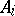,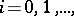where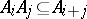for any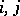. As a result, the additive group of a graded algebra (considered as a module over the ring of integers) is a positively graded module. As an example of a graded algebra one can quote the algebraof polynomials over a field, whereis the subspace generated by the monomials of degree(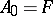). One can also define a graded algebrain a more general manner as an algebra whose additive group can be represented as a direct sum of groups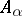, whereruns through a certain commutative semi-groupand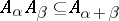for any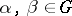. The concept of a filtered algebra is closely connected with that of a graded algebra. It is in fact possible to define, in a natural manner, an ascending filtration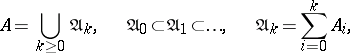on each graded algebra. Conversely, ifis a filtered algebra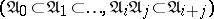, then one defines the graded algebra(where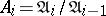,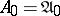), which is called the graded algebra associated with. A graded ring is defined in a similar manner.

For an arbitrary groupone may define a gradation of typeon an algebra, i.e.where each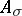is an additive subgroup ofand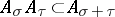for all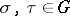. The group algebra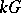over the field, as well as crossed products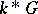defined by a group morphismand a-cocycle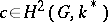, are examples of-graded algebras (see also Cross product). The use of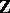-gradations that are not necessarily positive gradations allows one to consider the graded rings associated to-adic filtrations on a ring; for an idealofthe-adic filtration is given by a descending chain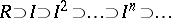, and then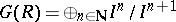, where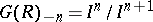is negatively graded.